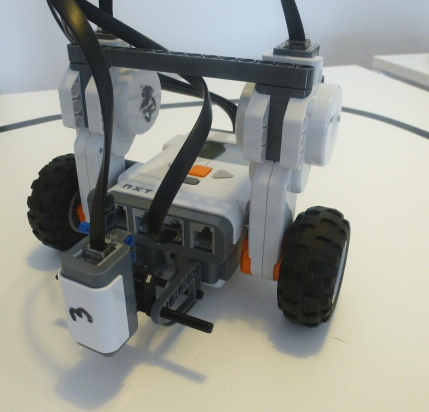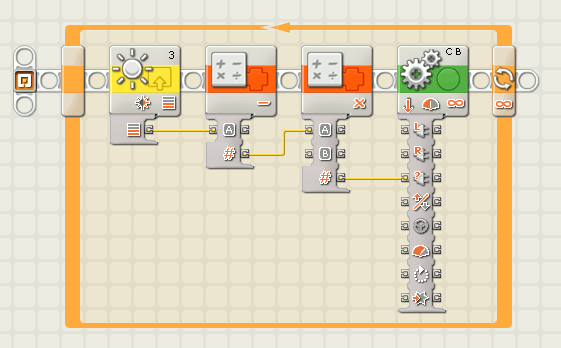# NXT Line Follower with Steering Control

There are many ways to program the NXT robot so it can follow a line. This article presents how to achieve line following using the steering option of the motor block in Lego's G-Code.## The control logic

Lego's tutorial shows a "Bang-Bang" control where the robot either turns left or right depending if the sensor is on a black or white surface. While being able to follow the line, the robot using this algorithm looks a bit drunk.A solution that produces a much better solution is the Fuzzy Logic approach presented earlier. However, only a few students at the primary school level are able to understand and program this algorithm.

A much simpler solution is to use the steering control of the NXT Motor Block. If the block receives a steering value of zero, the robot goes straight on. For positive values, it will turn to one side and for negative values to the other side. We want the robot to go straight on when it is exactly on the edge of the black line. Thus, the value the sensor reads when on the edge needs to be subtracted from the current sensor value.

Most of the time, the different sensor readings between the black line and the white surface are relatively small. The produced steering is not enough to keep the robot on the line. To solve that problem, we amplify the value obtained from the subtraction with a constant factor. The best value needs to be found through experimentation.

## The robot

The robot can be any type of robot that has one motor for each wheel. The light sensor needs to be placed in front of the robot, pointing to the surface at a 90° angle. The distance between the sensor and the surface should be around 2 cm. The robot used in the video is based on the "Simple Hello World NXT Robot" presented earlier.

## Building the program step by step

The whole program is implemented in NXT-G Code. You only need 5 different blocks to implement the algorithm.

1. Place a loop that runs forever.
2. Inside the loop, put a sensor reading block, two math blocks and a motor block. The math blocks can be found in the advanced menu.
3. For the sensor block, select "light sensor -reflected light" and set the correct port (where you connected your sensor).
4. In the first math block, select subtraction. Make the inputs and outputs of the blocks visible by clicking on the lower edge of each block. Connect the output of the sensor block to input "A" of the first math block. Manually enter the experimental value that has to be subtracted (around 40*) in the field for "B".
5. Connect the output of the first math block to input "A" of the second math block. Select "multiplication" as the operation and enter the multiplication factor (experimental, probably between 2 and 4) in the field for "B".
6. Connect the result of the second math block to the motor control blocks steering input. Make sure you select the correct ports as well as the right direction depending on your robot. For the speed, lower values will usually work better. Start with 50% power and increase it step by step.

* The best way to obtain the value to be subtracted in the first math block is the following: First put the robot on the white surface and note the value for white (Use the "View function of the NXT). Then put the robot on the black line and note the value obtained for the line. Finally add the two values and divide them by to (to build the average value). This is the number that you should put in case "B" of the first math block.